﻿ Inverse Trigonometric Function: Arctangent

# Inverse Trigonometric Function: Arctangent

• PRACTICE (online exercises and printable worksheets)

Before studying this section, you are encouraged to read Trying to ‘Undo’ Trigonometric Functions.

This section is a copy of Inverse Trigonometric Function: Arcsine, with appropriate changes.
If you've mastered the arcsine section, then this one should be quick and easy!

 For a function to have an inverse, each output must have exactly one corresponding input. Thus, only one-to-one functions have inverses. The tangent function doesn't have a true inverse, because the tangent function is not one-to-one. So, to try and define an ‘inverse tangent function’, we do the best we can. We throw away most of the tangent curve, leaving us with a piece that has three properties: the piece is one-to-one (and hence has an inverse) the piece covers all the outputs from the tangent function (the interval $\,(-\infty,\infty)\,$) the piece is close to the origin The green piece shown at right satisfies all three of these properties. This green piece is the restriction of the tangent curve to the interval $\,(-\frac{\pi}{2},\frac{\pi}{2})\,$. The function that the mathematical community calls ‘the inverse tangent function’ is not actually the inverse of the tangent function, because the tangent function doesn't have a true inverse. Instead, the ‘inverse tangent function’ is the inverse of this green piece of the tangent curve. Several Cycles ofthe Graph of the Tangent Function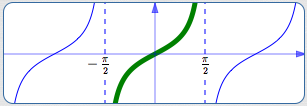The tangent function isn't one-to-one; it doesn't pass a horizontal line test. So, it doesn't have a true inverse. To define an ‘inverse tangent function’,we do the best we can. Throw away most of the curve—leave only the green part. This green part is one-to-one. This green part does have an inverse. The inverse of this green part is what the mathematical community calls ‘the inverse tangent function’.

The arctangent function (precise definition below) is the best we can do in trying to get an inverse of the tangent function.
The arctangent function is actually the inverse of the green piece shown above!

Here's a ‘function box’ view of what's going on:
 The tangent function takes a real number (excluding $\,\frac{\pi}{2} + k\pi\,$ for integers $\,k\,$) as an input. It gives a real number output. For example (as below), the output $\,0.5\,$ might come from the tangent function.When we try to use the tangent function box ‘backwards’, we run into trouble. The output $\,0.5\,$ could have come from any of the inputs shown.However, when we use the green piece of the tangent curve,the problem is solved! Now, there's only one input that works. (It's the value of the green $\,\color{green}{x}\,$.) Observe that $\,\color{green}{x}\,$ is in the interval $\,(-\frac{\pi}2,\frac{\pi}2)\,$.It's a bit of a misnomer, but the arctangent function (precise definition below) is often referred to as the ‘inverse tangent function’.
A better name would be something like ‘the inverse of an appropriately-restricted tangent function’.
(It's no surprise, however, that people don't say something that long and cumbersome.)

So, what exactly is $\,\arctan 0.5\,$?

$\,\arctan 0.5\,$ is the number in the interval $\,(-\frac{\pi}{2},\frac{\pi}{2})\,$ whose tangent is $\,0.5\,$

## What exactly IS $\,\arctan x\,$?

More generally, let $\,x\,$ be any real number.
Then:

$\,\arctan x\,$ is the number in the interval $\,(-\frac{\pi}{2},\frac{\pi}{2})\,$ whose tangent is $\,x\,$

In my own mind (author Dr. Carol Burns speaking here), the words I say are:

$\,\arctan x\,$ is the number between $\,-\frac{\pi}{2}\,$ and $\,\frac{\pi}{2}\,$ whose tangent is $\,x\,$
I personally know the endpoints are not included, so this doesn't confuse me.
However, the word ‘between’ is ambiguous—it can include the endpoints or not, depending on context.
It can be clarified by saying:
$\,\arctan x\,$ is the number between $\,-\frac{\pi}{2}\,$ and $\,\frac{\pi}{2}\,$ (not including the endpoints)
whose tangent is $\,x\,$
... but then it loses its simplicity. Ah—issues with language. Choose words that work for you!

## Precise Definition of the Arctangent Function

The precise definition of the arctangent function follows.
It can look a bit intimidating—the notes following the definition should help.

DEFINITION the arctangent function, denoted by  $\,\arctan\,$  or  $\,\tan^{-1}\,$
Let $\,x\,$ be a real number.

Using the notation ‘$\,\arctan\,$’ for the arctangent function: $$y = \arctan x\ \ \ \ \ \text{if and only if}\ \ \ \ \bigl(\ \tan y = x\ \ \text{AND}\ \ -\frac{\pi}{2} \lt y\lt \frac{\pi}{2}\ \bigr)$$
Using the notation ‘$\,\tan^{-1}\,$’ for the arctangent function: $$y = \tan^{-1} x\ \ \ \ \ \text{if and only if}\ \ \ \ \bigl(\ \tan y = x\ \ \text{AND}\ \ -\frac{\pi}{2} \lt y\lt \frac{\pi}{2}\ \bigr)$$

## Notes on the Definition of the Arctangent Function:

• UNDERSTANDING THE DEFINITION:
The definition precisely answers the question: ‘What is the number $\,\arctan x\,$?’
Here's how:
• Recall that ‘ if and only if ’ means the same thing as is equivalent to.
Equivalent sentences have identical truth values:
if one is true, so is the other;   if one is false, so is the other.
• Thus, if the sentence ‘$\,y = \arctan x\,$’ is true,
then the compound sentence   ‘$\,\tan y = x\ \ \text{AND}\ \ -\frac{\pi}{2} \lt y\lt \frac{\pi}{2}\,$’   must also be true.
• If   ‘$\,y = \arctan x\,$’   is true, then ‘$\,y\,$’ is just another name for ‘$\,\arctan x\,$’.
• If   ‘$\,y = \arctan x\,$’   is true, then two things must be true about $\,\arctan x\,$ (i.e., $\,y\,$):
• $\,\tan \overbrace{y}^{\arctan x} = x\,$:   its tangent must be $\,x\,$
• $\,-\frac{\pi}{2} \lt \overbrace{y}^{\arctan x}\lt \frac{\pi}{2}\,$:   it must be in the interval $\,(-\frac{\pi}{2},\frac{\pi}{2})\,$
• Combining these ideas:
$$\overbrace{\strut y = \arctan x}^{\text{How does a number get to be called \,\arctan x\,?}}\ \ \ \ \ \overbrace{\strut \text{if and only if}}^{\text{The answer is:}}\ \ \ \ \bigl(\ \overbrace{\strut \tan y = x}^{\text{by having its tangent equal to \,x\,}}\ \ \overbrace{\strut \text{AND}}^{\text{and}}\ \ \overbrace{\strut \ -\frac{\pi}{2} \lt y\lt \frac{\pi}{2}\ \ }^{\text{by being in the interval \strut \,(-\frac{\pi}{2},\frac{\pi}{2})\,}}\ \bigr)$$
• That is:
$\,\arctan x\,$ is the number in the interval $\,(-\frac{\pi}{2},\frac{\pi}{2})\,$ whose tangent is $\,x\,$
• FORMAL NAME VERSUS FUNCTION NOTATION ABBREVIATIONS:
The formal name of the function being discussed in this section is ‘arctangent’.
It is pronounced ARC-tan-gent.

When using function notation, ‘arctangent’ is abbreviated as ‘$\,\arctan\,$’.
It is pronounced the same as ‘arctangent’.
Thus, ‘$\,\arctan x\,$’ is read aloud as ‘arctangent of $\,x\,$’.

An alternate notation for the arctangent function is ‘$\,\tan^{-1}\,$’.
This alternate notation is modeled on the standard notation for inverse functions:
if $\,f\,$ is one-to-one, then its inverse is called $\,f^{-1}\,$.

The function notation ‘$\,\tan^{-1} x\,$’ can be read aloud as ‘arctangent of $\,x\,$’ or ‘the inverse tangent of $\,x\,$’.
DON'T read ‘$\,\tan^{-1} x\,$’ as ‘tangent to the negative one of $\,x\,$’!
There is no reciprocal operation going on here—it's just standard notation for an inverse function.
• CONVENTION FOR MULTI-LETTER FUNCTION NAMES:
Since both ‘$\,\arctan\,$’ and ‘$\,\tan^{-1}\,$’ are multi-letter function names, the standard convention applies:
the parentheses that typically hold the input can be removed, if there is no possible confusion about order of operations.

Thus, you usually see   ‘$\,\arctan x\,$’   and   ‘$\,\tan^{-1} x\,$’   (no parentheses),
instead of the more cumbersome   ‘$\,\arctan (x)\,$’   and   ‘$\,\tan^{-1} (x)\,$’   (with parentheses).
• FUNCTION NAME VERSUS OUTPUT FROM THE FUNCTION:
The function name is ‘$\,\arctan\,$’.
The number ‘$\,\arctan x\,$’ is the output from the function ‘$\,\arctan\,$’ when the input is $\,x\,$.

Similarly, the function name is ‘$\,\tan^{-1}\,$’.
The number ‘$\,\tan^{-1} x\,$’ is the output from the function ‘$\,\tan^{-1}\,$’ when the input is $\,x\,$.
• PREFERRED NOTATION:
Since the tangent function does not have a true inverse,
this author believes the notation ‘$\,\tan^{-1}\,$’ is misleading and lends itself to errors.
This author strongly prefers the notation ‘$\,\arctan\,$’.
Inputs to trigonometric functions can be viewed as real numbers (radian measure) or degrees.
For example, $\,\tan \frac{\pi}{4} = 1\,$:   here, $\,\frac{\pi}{4}\,$ is radian measure.
Equivalently, $\,\tan 45^\circ = 1\,$:   here, $\,45^\circ\,$ is degree measure.

Here's what the definition of arctangent looks like, using degree measure instead of radian measure: $$y = \arctan x\ \ \ \ \ \text{if and only if}\ \ \ \ \bigl(\ \tan y = x\ \ \text{AND}\ \ -90^\circ \lt y\lt 90^\circ\ \bigr)$$
$\,\arctan x\,$ is the angle in the interval $\,(-90^\circ,90^\circ)\,$ whose tangent is $\,x\,$
• CALCULATOR SKILLS:
If a calculator is in degree mode, then $\,\arctan x\,$ is reported in degrees.
If a calculator is in radian mode, then $\,\arctan x\,$ is reported in radians.
• GRAPH OF THE ARCTANGENT FUNCTION:
For a one-to-one function $\,f\,$, the graph of its inverse, $\,f^{-1}\,$, is found by reflecting the graph of $\,f\,$ about the line $\,y = x\,$.
Below, this technique is used to construct the graph of the arctangent function:

 Here's the piece of the tangent curve that is used to define the arctangent function: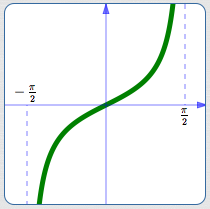domain:   $\,(-\frac{\pi}{2},\frac{\pi}{2})\,$ range:   $\,(-\infty,\infty)\,$ Here's the same curve, together with its reflection about the line $\,\color{red}{y = x}\,$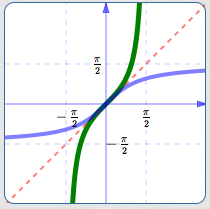The graph of the arctangent function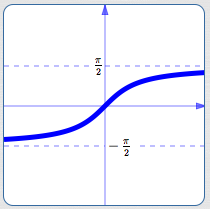domain:   $\,(-\infty,\infty)\,$ range:   $\,(-\frac{\pi}{2},\frac{\pi}{2})\,$ Notice that the domain and range of a function and its inverse are switched! The domain of one is the range of the other. The range of one is the domain of the other.
• RELATIONSHIP BETWEEN THE TANGENT AND ARCTANGENT FUNCTIONS:
For a one-to-one function $\,f\,$ and its inverse $\,f^{-1}\,$, there is a simple ‘undoing’ relationship between the two:
• $f^{-1}\bigl(f(x)\bigr) = x\,$ for all $\,x\,$ in the domain of $\,f\,$:
the function $\,f\,$ does something, and $\,f^{-1}\,$ undoes it
• $f\bigl(f^{-1}(x)\bigr) = x\,$ for all $\,x\,$ in the range of $\,f\,$:
the function $\,f^{-1}\,$ does something, and $\,f\,$ undoes it
Since the tangent and arctangent functions are not true inverses of each other,
the relationship between them is a bit more complicated.

 Here's the direction where they do ‘undo’ each other nicely: start with a number, first apply the arctangent function, then apply the tangent function, and end up right where you started. Here are the details: For all $\,x\in \Bbb R\,$, $$\tan(\arctan x) = x$$ start with $\,\color{red}{x}\in \Bbb R\,$ the arctangent function takes $\,\color{red}{x}\,$ to $\,\color{green}{\arctan x}\,$ in the interval $\,(-\frac{\pi}2,\frac{\pi}2)\,$ the tangent function takes $\,\color{green}{\arctan x}\,$ back to $\,\color{red}{x}\,$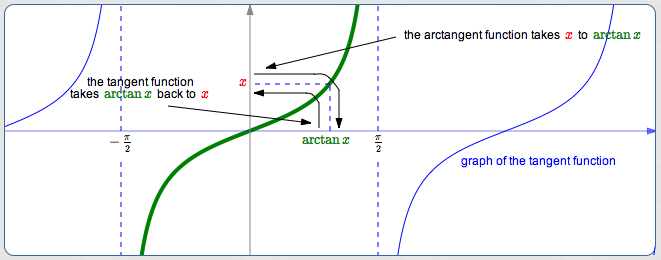Here's the direction where they don't necessarily ‘undo’ each other nicely: start with a number, first apply the tangent function, then apply the arctangent function. If the number you started with is outside the interval $\,(-\frac{\pi}2,\frac{\pi}2)\,$, then you don't end up where you started! Here are the details: For all $\,x\in (-\frac{\pi}2,\frac{\pi}2)\,$, $$\arctan(\tan x) = x$$ (See the top graph at right.) For all $\,x\,$ in the domain of the tangent function but not in $\,(-\frac{\pi}2,\frac{\pi}2)\,$, $$\arctan(\tan x) \ne x$$ (See the bottom graph at right.)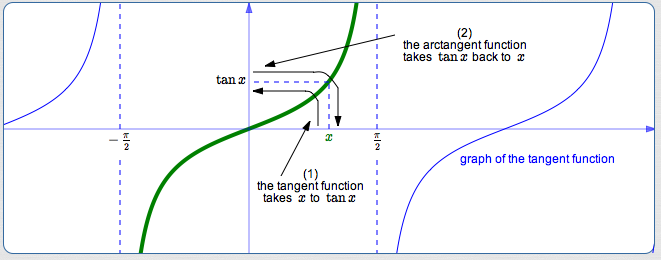## Example: Find the Exact Value of $\,\arctan(-1/\sqrt 3)\,$ (use both the unit circle and a special triangle)

 Using the degree definition: $\,\arctan (-1/\sqrt 3)\,$ is the angle between $\,-90^\circ\,$ and $\,90^\circ\,$ whose tangent is $\,-\frac{1}{\sqrt 3}\,$. As needed, review information about the size and sign of the tangent function. Draw a unit circle. Since we want an angle between $\,-90^\circ\,$ and $\,90^\circ\,$ whose tangent is negative, the angle is in quadrant IV. Since we want an angle whose tangent has size $\,\frac{1}{\sqrt 3} \approx 0.58\,$, make the red segment have this length. The (negative) angle shown is therefore $\,\arctan(-1/\sqrt 3)\,$. Does any special triangle tell us an acute angle whose tangent is $\,\frac{1}{\sqrt 3}\,$? Yes! The tangent of $\,30^\circ\,$ is $\,\frac{1}{\sqrt 3}\,$. Thus, $\,\arctan(-1/\sqrt{3}) = -30^\circ\,$. Using radian measure, $\,\arctan(-1/\sqrt{3}) = -\frac{\pi}6\,$.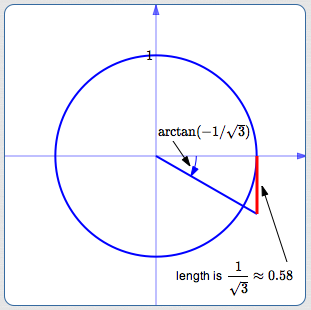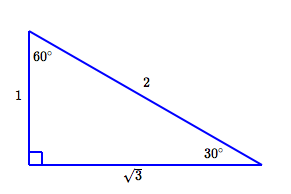Master the ideas from this section